# C++ Program to Find the Area and Perimeter of a Triangle

In this article, you will learn and get code for the area and perimeter of a triangle in C++ programming. There are many approaches used here to find the area and perimeter of a triangle. Here is the list:

### What Should You Do Before the Program?

If, before starting the program, you are not aware of triangle types and their related formula, you can refer to Area and Perimeter of Triangle Formula for all the necessary details.

## In C++, find the area of a triangle based on its base and height

This program finds the area of a triangle based on its base and height values. The question is, "Write a program in C++ to find the area of a triangle with base and height entered by the user at run-time." Here is its answer.

```#include<iostream>
using namespace std;
int main()
{
float b, h, area;
cout<<"Enter Base length of Triangle: ";
cin>>b;
cout<<"Enter Height length of Triangle: ";
cin>>h;
area = 0.5*b*h;
cout<<"\nArea = "<<area;
cout<<endl;
return 0;
}```

This program was built and runs under the Code::Blocks IDE. Here is its sample run:Now enter the value of the base and height of the triangle to find and print its area as shown in the output given below: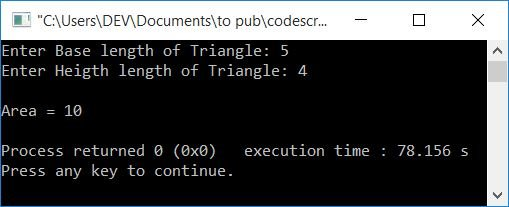## In C++, find the area of a triangle using Heron's Formula

This program uses Heron's formula to calculate the area of a triangle. Heron's formula is used when the lengths of all three sides are given:

```#include<iostream>
#include<cmath>
using namespace std;
int main()
{
float a, b, c, s, area;
cout<<"Enter Length of Three Sides (one by one): ";
cin>>a>>b>>c;
s = (a+b+c)/2;
area = sqrt(s*(s-a)*(s-b)*(s-c));
cout<<"\nArea = "<<area;
cout<<endl;
return 0;
}```

Here is a sample run with user input of 5, 4, and 3 as values for the triangle's three sides: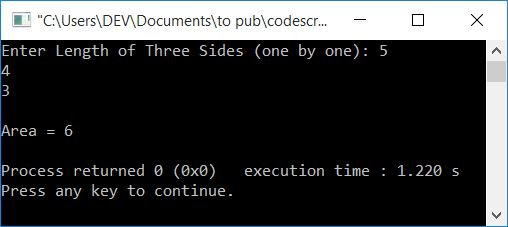Note: The function sqrt() returns the square root of the value passed as its argument. For instance, sqrt(25) returns 5.This function is defined in the cmath header file.

## In C++, find the perimeter of a triangle based on its three side lengths

Now let's create a program to find the perimeter of a triangle using the values of its three sides given by the user at run-time:

```#include<iostream>
using namespace std;
int main()
{
float a, b, c, per;
cout<<"Enter Length of First Side: ";
cin>>a;
cout<<"Enter Length of Second Side: ";
cin>>b;
cout<<"Enter Length of Third Side: ";
cin>>c;
per = a+b+c;
cout<<"\nPerimeter = "<<per;
cout<<endl;
return 0;
}```

Here is the initial snapshot of the sample run: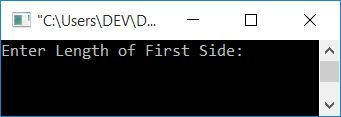Now supply the length of all three sides of the triangle, say 5, 4, and 3, and then press the ENTER key to see the following output: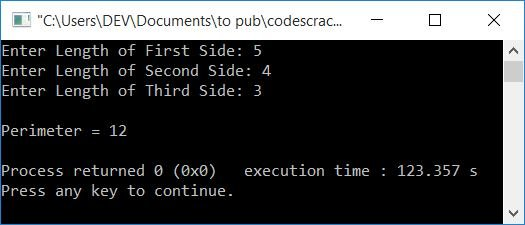### In C++, find the perimeter of all three types of triangles based on the user's choice

Here is another program for calculating the perimeter of a triangle. This is a menu-driven program that provides the user with four options to perform the task according to his or her choice:

```#include<iostream>
using namespace std;
int main()
{
float a, b, c, per;
int ch;
do
{
cout<<"1. Equilateral Triangle\n";
cout<<"2. Isosceles Triangle\n";
cout<<"3. Scalene Triangle\n";
cout<<"4. Exit\n";
cin>>ch;
switch(ch)
{
case 1:
cout<<"\nEnter Side Length: ";
cin>>a;
per = 3*a;
break;
case 2:
cout<<"\nEnter First Length (of Equal Sides): ";
cin>>a;
cout<<"Enter Second Length: ";
cin>>b;
per = 2*a+b;
break;
case 3:
cout<<"\nEnter Length of all 3 Sides: ";
cin>>a>>b>>c;
per = a+b+c;
break;
case 4:
break;
default:
cout<<"\nWrong Choice!";
continue;
}
if(ch>=1 && ch<=3)
cout<<"\nPerimeter = "<<per<<"\n\n";
}while(ch>=1 && ch<=3);
cout<<endl;
return 0;
}```

Here is its sample run: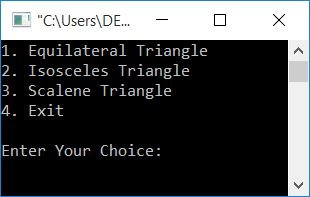Now provide your choice according to the following information:

• If all the sides of a triangle are the same length, then it is an equilateral triangle.
• If two sides are the same length, then it is an isosceles triangle.
• And if none of the sides are the same length or all three sides are different lengths, then it is a scalene triangle.

Now, if you have a triangle whose two sides are the same length, press 2 as your choice, enter the first length (the length of the side that has another duplicate side of the same length), and then enter the second length of the triangle. Press the ENTER key to see its perimeter as output.

After printing the perimeter, it will again display the menu to choose and operate for another triangle or for the same triangle with a different length, until and unless you press 4 to exit from it.## In C++, find the area and perimeter of a triangle using the function

This program finds both the area and perimeter of a triangle using a user-defined function. Area and perimeter are calculated based on three sides' input:

```#include<iostream>
#include<cmath>
using namespace std;
float areaOfTriagle(float, float, float);
float perOfTriangle(float, float, float);
int main()
{
float a, b, c, area, per;
cout<<"Enter Length of all Three Sides: ";
cin>>a>>b>>c;
area = areaOfTriagle(a, b, c);
cout<<"\nArea = "<<area;
per = perOfTriangle(a, b, c);
cout<<"\nPerimeter = "<<per;
cout<<endl;
return 0;
}
float areaOfTriagle(float a, float b, float c)
{
float s, ar;
s = (a+b+c)/2;
ar = sqrt(s*(s-a)*(s-b)*(s-c));
return ar;
}
float perOfTriangle(float a, float b, float c)
{
return (a+b+c);
}```

A sample run of the above program is given below: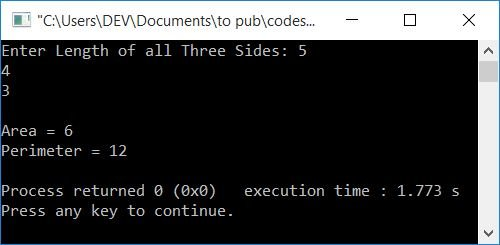## In C++, using classes and objects, calculate the area and perimeter of a triangle

This program uses class and object to find and print the area and perimeter of a triangle with three sides:

```#include<iostream>
#include<cmath>
using namespace std;
class CodesCracker
{
private:
float a, b, c, s, ar;
public:
void getData();
float areaOfTriagle();
float perOfTriangle();
};
void CodesCracker::getData()
{
cout<<"Enter Length of all Three Sides: ";
cin>>a>>b>>c;
}
float CodesCracker::areaOfTriagle()
{
s = (a+b+c)/2;
ar = sqrt(s*(s-a)*(s-b)*(s-c));
return ar;
}
float CodesCracker::perOfTriangle()
{
return (a+b+c);
}
int main()
{
CodesCracker c;
float area, perimeter;
c.getData();
area = c.areaOfTriagle();
cout<<"\nArea = "<<area;
perimeter = c.perOfTriangle();
cout<<"\nPerimeter = "<<perimeter;
cout<<endl;
return 0;
}```

It will produce the same output as the previous program.

C++ Quiz

« Previous Program Next Program »